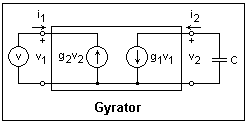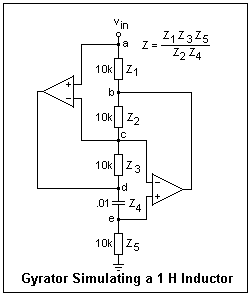## The Gyrator

Inductance without MagnetismThe gyrator is a 2-port network containing two current sources controlled by the terminal voltages and oppositely directed, as shown inside the rectangular outline in the figure. Its two parameters g1, g2 are transconductances, units A/V. In the figure, it is shown fed by a voltage source v and loaded by a capacitor C. The circuit is easy to solve, giving i1 = (g1g2/jωC)v, or Z = jω(C/g1g2) = jωL, so a capacitive reactance is converted to an inductive reactance. The circuit effectively inverts its load impedance, changing 1/jωC into jωC.No passive network can function as a gyrator, but with the help of operational amplifiers it is not difficult to build one. The figure shows a circuit that is sometimes called a generalized impedance converter (GIC). The circuit is shown with component values that cause it to simulate an inductance of 1 H. It is not difficult to analyze this circuit. The voltages at nodes a, c and d are equal if the feedback is working, and equal to the applied voltage. Now all the voltages can be found by starting at the lowest resistor and calculating the currents working upwards, remembering that the op-amp input currents are zero. Finally, the impedance the circuit presents to the source is just the applied voltage divided by the current. The expression is shown in the figure. Nothing in the circuit is connected to ground except the lowest impedance, so the ground connection is not essential.

The circuit was tested by applying a sinusoidal voltage of various frequencies, while measuring the voltage across the circuit and the current through it with the AC scales of two DMM's. The waveforms are sinusoidal, so the meters read properly. Between about 500 Hz and 7000 Hz, the circuit did simulate a 1 H inductor quite well. At lower frequencies, the reactance of the capacitor becomes large, and the op-amp outputs tend to saturate. At higher frequencies, the capacitive reactance becomes small and overloads the op-amps. In both cases the feedback becomes inaccurate, and the apparent inductance rises. The capacitor must be properly chosen for the frequency range desired. Note that the capacitor may also be in the position of Z2. I used LM833 and LM385 dual op-amps with essentially the same results for either.

To observe the time-domain behavior, apply a square wave to the circuit through a 10k resistor. Use a unity-gain buffer to avoid loading the signal generator so the square wave does not droop. Observe the applied voltage as well as the voltage across the circuit with an oscilloscope. The voltage across the circuit decreased exponentially to zero with the expected time constant of L/R = 0.0001 s, a very pretty result. Unlike a normal inductor, the current that this circuit can absorb is limited. To draw the input voltage to zero, the lower op-amp output voltage must sink to 10k times the input current. Otherwise, the output will saturate and the feedback will fail. This would occur if we used a 1k resistor instead of a 10k resisor as the R in our RL circuit, trying to get a time constant of 1 ms. This limitation must be carefully watched in applying this circuit, and the component values should be carefully chosen to eliminate it. This is, indeed, a remarkable circuit that presents inductance without magnetic fields.Related to the gyrator is the Negative Impedance Converter, illustrated at the right. An easy analysis shows that it converts an impedance Z into its negative, -Z. This will convert a capacitive reactance 1/jωC into the inductive reactance j(1/ωC). This is not a real inductance, since the reactance decreases as the frequency increases instead of increasing. The inductance is a function of frequency, not a constant. If you test the circuit shown, a voltage V applied to the input will cause a current V/10kΩ to flow out of the input terminal, instead of into it, as would occur with a normal resistance.

A 411 op-amp operates as desired with a resistive Z, but if you replace the resistance with an 0.01 μF capacitor, the circuit will oscillate (I measured 26.4 kHz), the op-amp output saturating in both directions alternately. When the op-amp was replaced by an LM748 with a (very large) compensation capacitor of 0.001 μF, the circuit behaved somewhat better. Using an AC voltmeter and milliammeter, the reactance varied from 16 kΩ at 1 kHz to 32 kHz at 500 Hz, showing that it does indeed decrease as the frequency increases. An investigation with the oscilloscope would verify that the reactance was inductive, with voltage leading the current. The waveform rapidly becomes a triangle wave at higher frequencies (because of the large compensation capacitance). It is clear that this circuit is not a practical one for converting a capacitance to an inductance, even if the strange frequency dependence is accepted.

### References

A. S. Sedra and K. C. Smith, Microelectronic Circuits, 2nd ed. (New York: Holt, Rinehart and Winston, 1987), pp. 40, 79, 111-113 .

P. Horowitz and W. Hill, the Art of Electronics, 2nd ed. (Cambridge: Cambridge Univ. Press, 1989, pp. 266, 281.